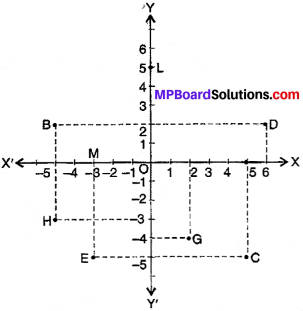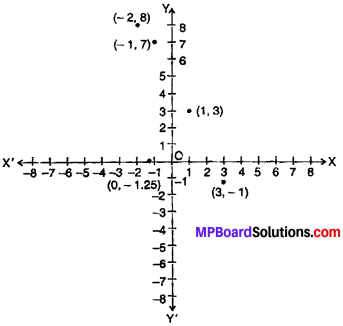# MP Board Class 9th Maths Solutions Chapter 3 Coordinate Geometry Ex 3.2

## MP Board Class 9th Maths Solutions Chapter 3 Coordinate Geometry Ex 3.2

Question 1.
Write the answer of each of the following questions:

1. What is the name of horizontal and the vertical lines drawn to determine the position of any point in the Cartesian plane?
2. What is the name of each part of the plane formed by these two lines?
3. Write the name of the point where these two lines intersect.

Solution:

1. The x – axis and the y – axis.
3. The origin.Question 2.
See figure and write the following:

1. The coordinates of B.
2. The coordinates of C.
3. The point identified by the coordinates (-3, -5)
4. The point identified by the coordinates (2, -4).
5. The abscissa of the point D.
6. The ordinate of the point H.
7. The coordinates of the point L.
8. The coordinates of the point M.Solution:

1. B → (- 5, 2 )
2. C → 4 (5, – 5 )
3. E
4. G
5. 6
6. – 3
7. L → (0, 5 )
8. M → (-3, 0 )Question 3.
In which quadrant or on which axis do each of the points (-2, 4), (3, -1), (-1, 0), (1, 2) and (-3, -5) lie? Verify your answer by locating them on the Cartesian plane.
Solution:

1. The point (-2, 4) lies in the II quadrant.
2. The point (3, -1) lies in the IV quadrant.
3. The point (-1, 0) lies on the negative x-axis.
4. The point (1, 2) lies in the I quadrant.
5. The point (-3, -5) lies in the III quadrant.Question 4.
Plot the points (x, y) given in the following table on the plane, choosing suitable units of distance on the axes.Solution:Plotting of points on Graph Paper:
Steps:

1. Draw a horizontal and vertical line mutually perpendicular to each other.
2. Mark the point of inter section of two lines as ‘O’. This represent origin and horizontal line XOX’ vertical line as YOY’.
3. The line XOX’ will show X – axis and vertical line YOY’ will Y – axis.
4. Choose a suitable scale and marks the points on X – axis and Y – axis.
5. Obtain the coordinates of the given point and mark point.